## Numpy基本操作——通用函数的操作

news/2023/6/8 0:15:03

# Numpy基本操作——通用函数的操作

### 文章目录

• Numpy基本操作——通用函数的操作
• 一、实验目的
• 二、实验内容
• 三、实验环境
• 四、实验步骤

Python 3.6.1以上

Jupyter

# 四、实验步骤

1.创建一维数组x，使用np.sin函数对x中所有元素求正弦值

``````x=np.arange(0,100,10,dtype=np.floating)
np.sin(x)
``````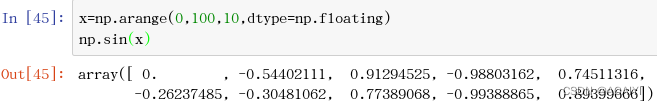2.创建二维数组b，使用np.cos函数对b数组中所有元素求余弦值

``````b=np.arange(1,10).reshape(3,3)
np.cos(b)
``````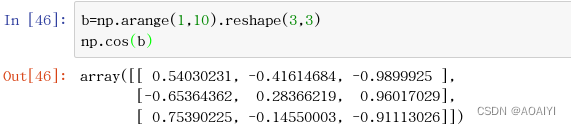3.使用np.round函数，对上面余弦结果数组元素进行四舍五入。

``````np.round(_)
``````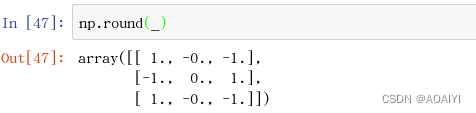4.使用numpy中的random.rand函数创建包含10个随机数的数组。

``````x=np.random.rand(10)*10
print(x)
``````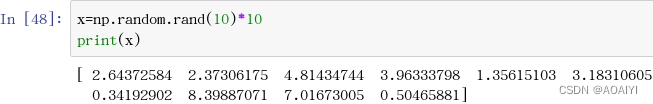5.使用np.floor函数对所有元素向下取整。

``````np.floor(x)
``````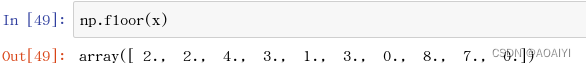6.使用np.ceil函数对所有元素向上取整。

``````np.ceil(x)
``````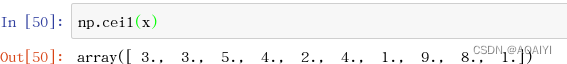7.使用np.absolute函数对-3取绝对值运算

``````np.absolute(-3)
``````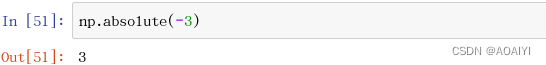8.使用np.absolute函数，对3+4j模运算

``````np.absolute(3+4j)
``````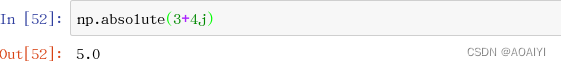9.使用isnan函数，判断是否为空

``````np.isnan(np.NAN)
``````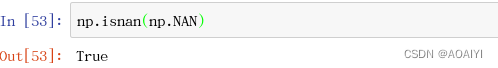10.使用np.sqrt函数对range(10)进行平方根运算

``````np.sqrt(range(10))
``````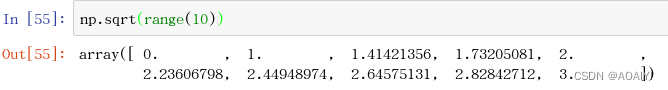11.创建一个数组a，使用np.mean函数求数组a的均值

``````a=np.array([1,2,6,3,4,5,8])
np.mean(a)
``````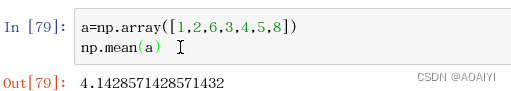12.使用np.average()函数，求range(1,5)的加权weights=range(4,0,-1)的平均值。

``````np.average(range(1,5),weights=range(4,0,-1))
``````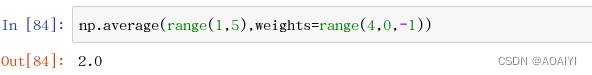13.创建一个数组a，使用np.var()函数，求数组a的方差值。

``````a=np.array([1,2,6,3,4,5,8])
np.var(a)
``````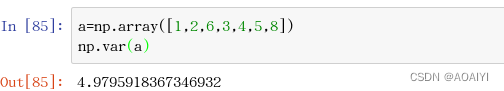14.创建一个数组a，使用np.std()函数，求数组a的标准差值。

``````a=np.array([1,2,6,3,4,5,8])
np.std(a)
``````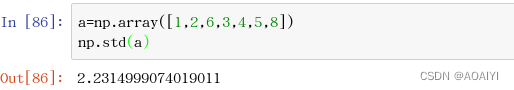15.点积dot()，创建两个数组a,b，计算两个数组的线性组合，一维上是对应元素相乘，多维可将a*b视为矩阵乘法

``````a=np.array((1,2,3))
b=np.array((4,2,1))
a.dot(b) #向量内积
np.dot(a,b)
``````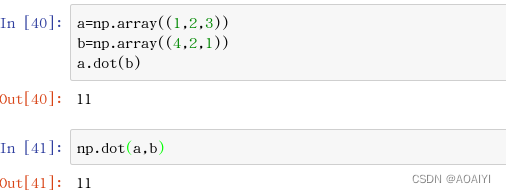### linux snort centos搭建入侵检测系统snort及问题总结与解答

linux系统centos搭建入侵检测系统snort及问题总结与解答 一、环境准备 1.安装Centos6&#xff08;安装选择开发环境&#xff0c;这样可以少装一些开发包&#xff09;&#xff0c;设置NAT获取&#xff0c;让系统可以上网&#xff0c;外加一台XP用于测试&#xff08;可用可不&am…